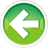# 5.x — Chapter 5 comprehensive quiz

Quick review

Always use parentheses to disambiguate the precedence of operators if there is any question or opportunity for confusion.

The arithmetic operators all work like they do in normal mathematics. The Modulus (%) operator returns the remainder from an integer division. Prior to C++11, beware of integer division and modulus using negative numbers.

The increment and decrement operators can be used to easily increment or decrement numbers. Avoid the postfix versions of these operators whenever possible.

Beware of side effects, particularly when it comes to the order that function parameters are evaluated. Do not use a variable that has a side effect applied more than once in a given statement.

Relational operators can be used to compare floating point numbers. Beware using equality and inequality on floating point numbers.

Logical operators allow us to form compound conditional statements.

Quiz time

Question #1

Evaluate the following:

a) (5 > 3 && 4 < 8)

Show Solution

b) (4 > 6 && true)

Show Solution

c) (3 >= 3 || false)

Show Solution

d) (true || false) ? 4 : 5

Show Solution

Question #2

Evaluate the following:

a) 7 / 4

Show Solution

b) 14 % 5

Show Solution

Question #3

Why should you never do the following:

a) int y = foo(++x, x);

Show Solution

b) float x = 0.1 + 0.1; if (x == 0.2) return true; else return false;

Show Solution

c) int x = 3 / 0;

Show SolutionO.1 -- Bit flags and bit manipulation via std::bitsetIndex5.7 -- Logical operators

### 3 comments to 5.x — Chapter 5 comprehensive quiz

•Speeds03

What I don't understand from the quiz is #4; whether or not i'm using 4 bit, 8 bit etc... How would I know that with 15 decimal, I should start of with "15 >= 8" instead of "15 >= 16". The same goes for 53 decimal (or any other number for that matter...

•Thomas

You must always think in bytes allocated for the given number, because that is the smallest unit that can be addressed in memory. If you allocated an int for it, that should mean 4 bytes (at least, on 32 bit architectures). In a case like this quiz, you only need to check whether the given number can be stored in 1 byte (8 bits, like a char or bool). If it's too big for that, then you must go up to 2 bytes (like short int). If it's still too big, then 4 bytes (int) and so on.

•zingmars

Woah, It's been a while since you actually posted something.
Is this something you made because you were bored, or can we expect more tutorials (C++11 for example) in the future?